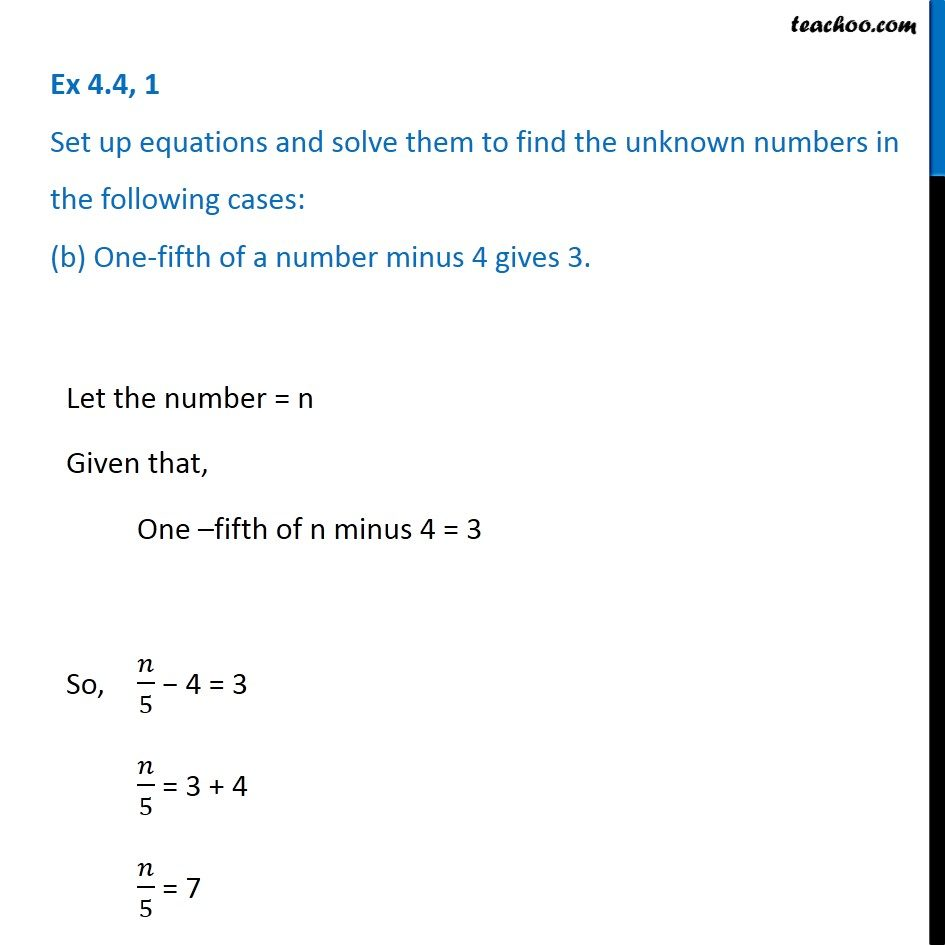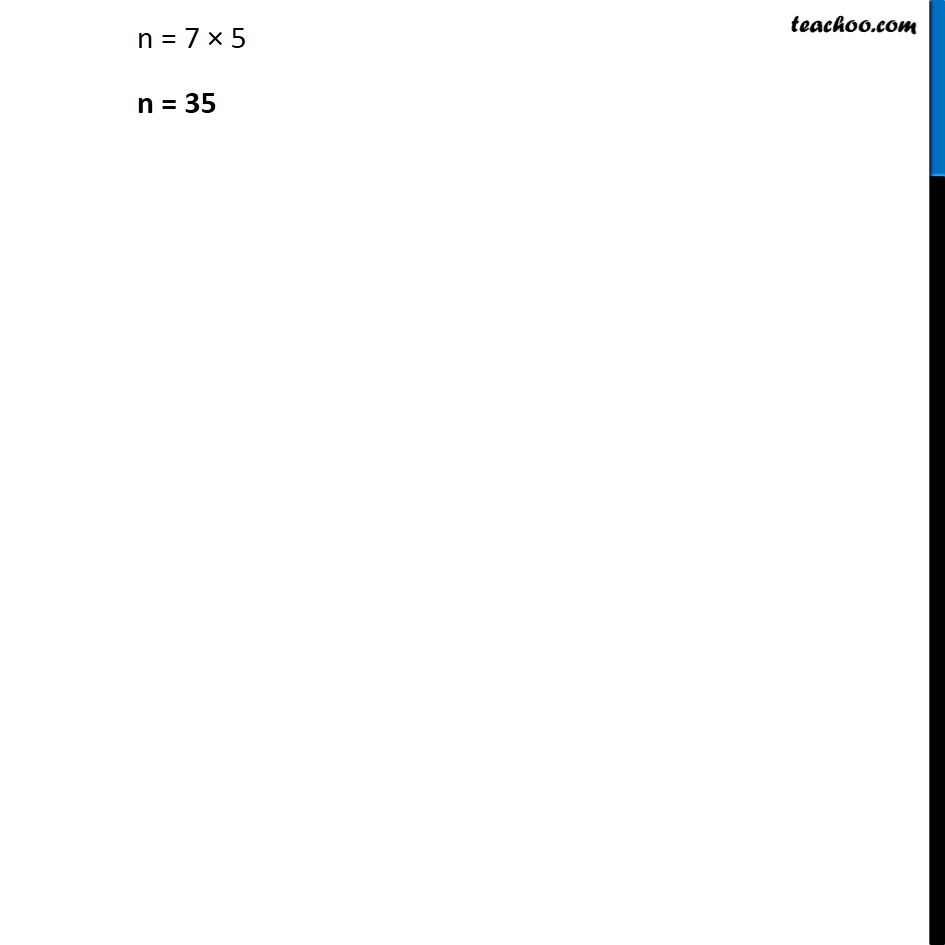Ex 4.4

Chapter 4 Class 7 Simple Equations
Serial order wiseIntroducing your new favourite teacher - Teachoo Black, at only ₹83 per month

### Transcript

Ex 4.4, 1 Set up equations and solve them to find the unknown numbers in the following cases: (b) One-fifth of a number minus 4 gives 3. Let the number = n Given that, One –fifth of n minus 4 = 3 So, 𝑛/5 − 4 = 3 𝑛/5 = 3 + 4 𝑛/5 = 7 Let the number = n Given that, One –fifth of n minus 4 = 3 So, 𝑛/5 − 4 = 3 𝑛/5 = 3 + 4 𝑛/5 = 7 Let the number = n Given that, One –fifth of n minus 4 = 3 So, 𝑛/5 − 4 = 3 𝑛/5 = 3 + 4 𝑛/5 = 7 n = 7 × 5 n = 35